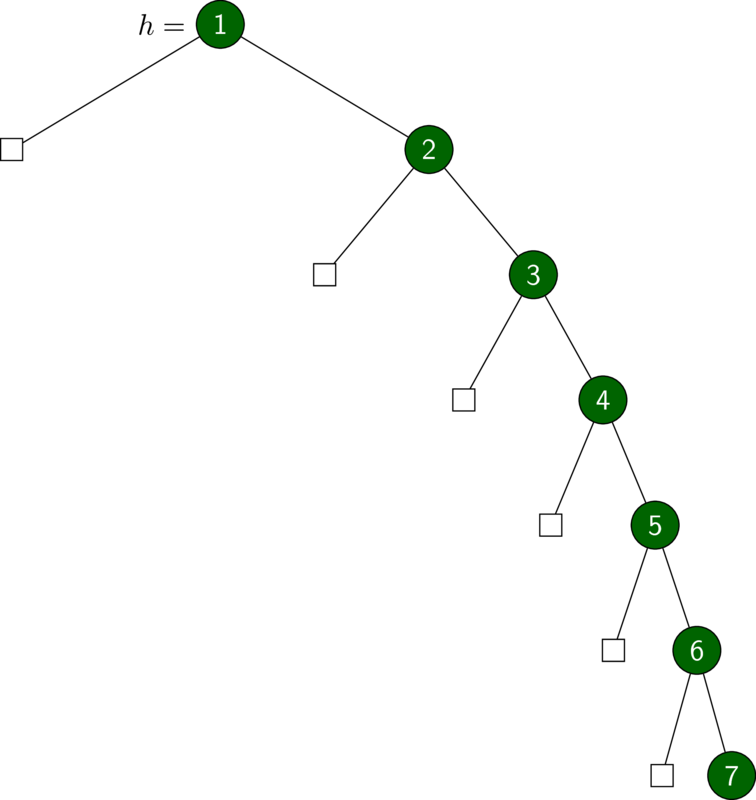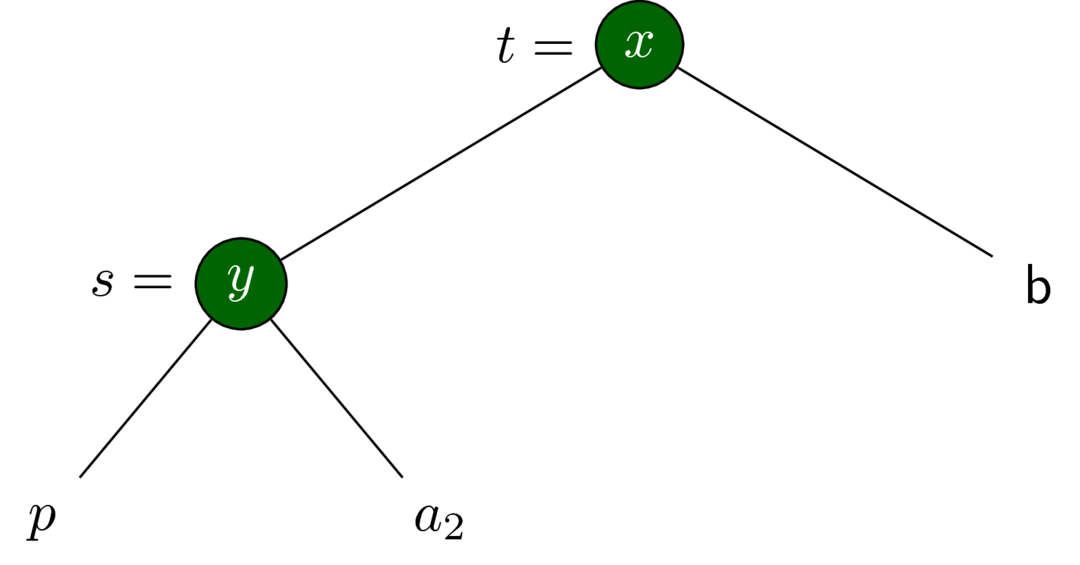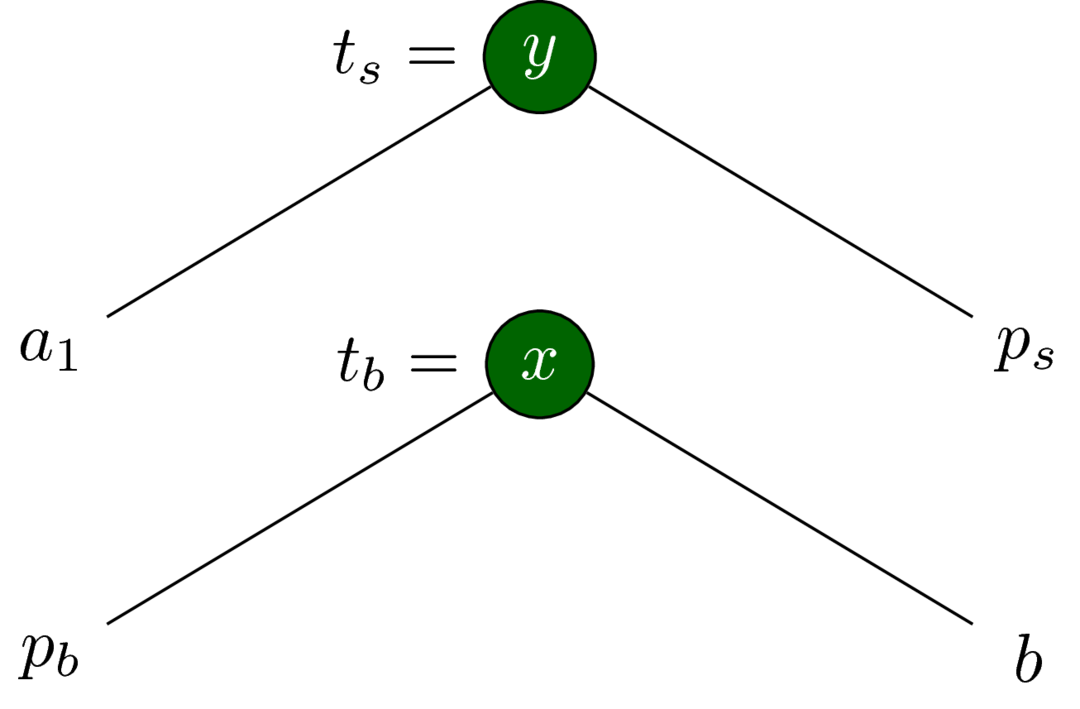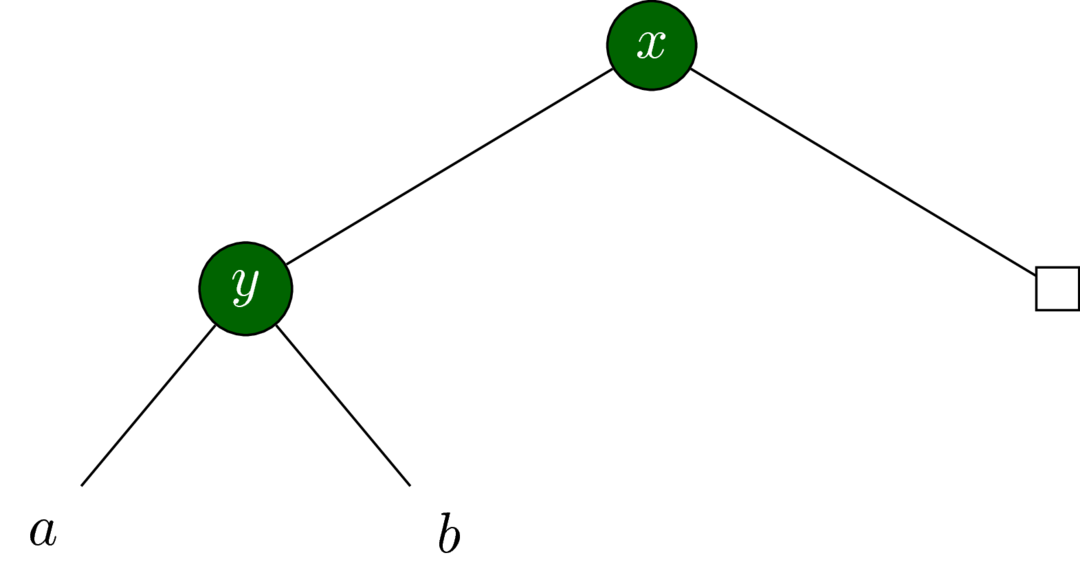# Okasaki's PFDS, Chapter 5

This post contains my solutions to the exercises in chapter 5 of Okasaki’s ‘Purely Functional Data Structures’. The latest source code can be found in my GitHub repo.

## Exercise 5.1

1. Implement deques.
2. Prove that each deque operation takes $$\mathcal O (1)$$ amortised time using the potential $\Phi (f, r) = \left| \left|f\right| - \left|r\right| \right|.$

## Solution 5.1

Item 1.

See source.

Item 2.

By symmetry, the costs of cons, head, and tail are (almost) identical to those of snoc, last, and init, respectively.

Consider cons. There is a constant number of actual steps and the potential can change by at most 1. Thus cons runs in constant amortised time.

Consider tail. Any tail which doesn’t empty f requires only one step and changes the potential by one for an amortised cost of $$\le 2$$. Any tail which does empty f requires $$1 + 2m + \delta$$ steps, where $$m := \left\lfloor \frac{r}{2} \right\rfloor$$, $$\left| r \right| = 2m + \delta$$. The linearity is due to the fact that it takes $$m$$ steps to split r in half, then $$m$$ more steps to reverse the other half. The change in potential is given by

\begin{align} \left|1 - (2m + \delta)\right| - \left|m - (2m + \delta - m)\right| &= 2m + 1 + \delta - \delta \\ &= 2m + 1 . \end{align}

Thus, the amortised cost is $$1 + 2m + \delta - 2m = 1$$, showing that tail runs in constant amortised time.

## Exercise 5.2

Prove that insert on binomial heaps runs in $$\mathcal O (1)$$ amortised time using the banker’s method.

## Solution 5.2

The credit invariant associates one credit to every binomial tree in the heap. Let $$k$$ be the number of calls to link made by a call to insert. A call to insert takes $$1 + k$$ actual steps. It initially adds a tree to the heap, gaining a credit, and each link removes a tree, spending a credit. Thus, the total amortised cost is $$(1+k) + 1 - k = 2$$.

## Exercise 5.3

Prove that the amortised costs of merge and deleteMin are still $$\mathcal O (\log n)$$.

## Solution 5.3

Let $$h_m$$, $$h_n$$ be binomial heaps with potentials $$m$$, $$n$$, respectively. We show that the amortised cost of merge is $$A(h_m, h_n) \le m+n$$. Let $$k$$ be the number of calls to link. The actual cost is bounded by $$m + n + k$$, since there can be at most $$m+n$$ recursive calls to merge and any call reaching the third conditional clause of merge will call link several times via insTree. We start with a potential of $$m+n$$, and each call to link reduces this by one, for an end potential of $$m+n-k$$. The change in potential is $$m + n - (m + n - k) = k$$. Thus, the amortised cost of merge is $$m+n+k -k = m+n$$.

Now we show that deleteMin is also logarithmic. We start with a heap $$h_n$$, which has potential $$n$$. There is an actual cost of at most $$n$$ to find the minimum binary tree, say of rank $$r$$. This leaves us with a heap of rank $$n-1$$. Then there is an actual cost of at most $$r$$ to reverse the list of children, making a heap of potential $$r$$. Merging these heaps then takes at most $$n + r - 1 + k$$ steps, where $$k$$ is the number of calls to link, which leaves us with a heap with potential $$n + r - 1 - k$$. This is a total of at most $$n + r + (n + r - 1 + k)$$ steps. The change in potential is $$n - (n + r - 1 - k) = 1 - r + k$$. Thus, the amortised cost of deleteMin is

$2n + 2r + k - 1 - (1 - r + k) = 2n + 3r - 2 .$

Note that this is indeed logarithmic since, if a heap has a tree of rank $$r$$, then it must have at least $$2^r$$ elements; that is, $$r = \mathcal O (\log n)$$.

## Splay Heaps

A splay heap is a BST that rebalances the tree using a partition function when performing update operations. However, we now allow the insertion of the same element multiple times since we are implementing a heap and not a set.h = foldr insert empty [1..7]insert 8 h

## Exercise 5.4

Implement smaller.

See source.

## Exercise 5.5

Prove that partition is logarithmic (in the zig-zag case).

## Solution 5.5

First we will need a modification to the lemma proved in the book.

Lemma

We have the inequality

$1 + \log x + \log y \le 2\log (x + y -1) .$

for all $$x \in \mathbb N_{\ge 2}$$, $$y \in \mathbb N_{\ge 1}$$.

Using the basic logarithmic identities, the above inequality is equivalent to $$2xy \le (x+y-1)^2$$. In other words, we must show that $$x^2 -2x + (y-1)^2 \ge 0$$ for $$x \ge 2$$, $$y \ge 1$$. The term with $$y$$ is non-negative. The remaining term $$x^2 -2x$$ is non-negative for any $$x \ge 2$$.We wish to analyse partition pivot t.Suppose partition pivot t outputs $$(t_s, t_b)$$.

Define $$(p_s, p_b)$$ as the output of partition pivot p. Note that $$\#t_s + \#t_b = \#t - 1$$, so that $$1 + \phi(t_s) + \phi(t_b) \le 2\phi(t)$$ by the lemma.

\begin{align} A (t) &= T (t) + \Phi (t_s) + \Phi (t_b) - \Phi (t) \\ &= 1 + T (p) + \Phi (t_s) + \Phi (t_b) - \Phi (t) \\ &= 1 + A (p) - \Phi (p_s) - \Phi (p_b) + \Phi (p) \\ &\qquad + \Phi (t_s) + \Phi (t_b) - \Phi (t) \\ &= 1 + A (p) - \Phi (p_s) - \Phi (p_b) + \Phi (p) \\ &\qquad + \phi (t_s) + \Phi (a_1) + \Phi (p_s) \\ &\qquad + \phi (t_b) + \Phi (p_b) + \Phi (b) \\ &\qquad - \phi (t) - \phi (s) - \Phi (b) - \Phi (a_1) - \Phi (p) \\ &= 1 + A (p) + \phi (t_s) + \phi (t_b) - \phi (t) - \phi (s) \\ &\le 2 + 2\phi (p) + \phi(t_s) + \phi(t_b) - \phi(t) - \phi(s) \\ &\le 2 + \phi(t) + \phi(s) + \phi(t_s) - \phi(t_b) - \phi(t) - \phi(s) \\ &\le 2 + \phi(t_s) + \phi(t_b) \\ &\le 1 + 2\phi(t) \end{align}

## Exercise 5.6

Prove that deleteMin also runs in logarithmic time.

## Solution 5.6

We prove that deleteMin runs in $$\mathcal O(3\log n)$$ amortised time. Note that $$\#a + (\#b + \#c) \le \#s_1$$ so that $$1 + \phi(a) + \phi(t_2) \le 2\phi(s_1)$$.

\begin{align} A(s_1) &= T(s_1) + \Phi(t_1) - \Phi(s_1) \\ &= 1 + T(a) + \Phi(t_1) - \Phi(s_1) \\ &= 1 + A(a) - \Phi(a') + \Phi(a) \\ &\qquad + \phi(t_1) + \phi(t_2) + \Phi(a') + \Phi(b) + \Phi(c) \\ &\qquad - \phi(s_1) - \phi(s_2) - \Phi(a) - \Phi(b) - \Phi(c) \\ &= 1 + A(a) + \phi(t_1) + \phi(t_2) - \phi(s_1) -\phi(s_2) \\ &\le 1 + \phi(a) + \phi(t_1) + \phi(t_2) \\ &\le \phi(t_1) + 2\phi(s_1) \\ &\le 3\phi(s_1) \end{align}

## Exercise 5.7

Write a sorting function that inserts elements into a splay tree and then performs an in order traversal of the tree dumping the elements into a list. Show that this function takes linear time in a sorted list.

## Solution 5.7

See source.

Let xs be a list of length $$n$$ in decreasing order. We can measure the complexity of sort xs by counting the number of calls to partition. Every time we call insert x h, we know that $$x > y$$ for all $$y$$ in h, so insert x h calls partition exactly once. The function sort xs makes a total of $$n$$ calls to insert and thus also $$n$$ calls to partition, showing that sort runs in $$\mathcal O (n)$$ time.

The argument for lists in increasing order is completely analogous.

## Pairing Heaps

A pairing heap is a heap-ordered multiway tree whose deleteMin operation merges the children in pairs.h = foldr insert empty [7, 6..1]deleteMin h

## Exercise 5.8

1. Write a function toBinary that converts pairing heaps to binary trees.

2. Reimplement pairing heaps using this new representation as binary trees.

3. Prove that deleteMin and merge still run in logarithmic amortised time in this new representation.

## Solution 5.8

Item 1.

See source.

The conversion from a pairing heap to a binary tree is explained in the book.For any binary tree derived from a pairing heap, $$x \le y, y_a, y_b$$ for all elements $$y_a, y_b$$ in the trees $$a, b$$, respectively. The right child of the root is empty. The values in $$b$$ are not related to $$y$$.

The invariant on a pairing heap T x cs is that x is no greater than any of the elements of its children in cs. This translates into the binary tree invariant that a node is no greater than any of its left descendants. That is, for T' x (T' y a b) c we have that $$x \le y, y_a, y_b$$ for all elements $$y_a, y_b$$ in the trees $$a, b$$, respectively. The value of $$x$$ bears no relation to the values in $$c$$.

We also maintain a second invariant: the right child of the root is empty.

Item 2.

See source.

Remember that the root of a binary tree representation of a pairing heap has no right child (it is empty). Thus we can forget about the right child without losing desired information.

Item 3.

We start with merge. Note that for any $$x, y \ge 2$$, we have $$\log (x+y) \le \log x + \log y$$. In particular, $$\#s_k \ge 2$$.

\begin{align} A (s_1, s_2) &= T (s_1, s_2) + \Phi (t_1) - \Phi (s_1) - \Phi (s_2) \\ &= 1 + \Phi(t_1) - \Phi(s_1) - \Phi(s_2) \\ &= 1 + \phi(t_1) + \phi(t_2) - \phi(s_1) - \phi(s_2) \\ &\le 2 + 2\phi(t_1) \\ &\le 2 + 2\log (\#s_1 + \#s_2) \\ &\le 2 + 2\log(\#s_1) + 2\log(\#s_2) \\ &\le 2 + 2\phi(s_1) + 2\phi(s_2) \end{align}

Now consider deleteMin. I was unable to find a nice solution by myself. The following comes from The Pairing Heap: A New Form of Self-Adjusting Heap. We reproduce their argument that the asymptotic cost of deleteMin is $$A(s_1) \le 2\phi(s_1) + 3$$.

There are at most $$2k+1$$ calls to merge, where $$k$$ is the number of children of the root of the pairing heap. The difficult part is calculating the potential increase, which we do in steps.

Lemma 1
Let $$x, y > 0$$ such that $$x + y \le 1$$. Then $$\log x + \log y \le -2$$.

Proof. This follows from the fact that $$xy \le x(1-x)$$, which has a maximum of $$\frac{1}{4}$$ at $$x = \frac{1}{2}$$.

Corollary

We have

$\log(x + y) - \log(y + z) \le 2 \log (x + y + z) - 2\log z - 2 ,$

for any $$x, y, z \ge 0$$.

Proof. By the lemma we have

\begin{align} \log(x + y) + \log z - 2\log (x + y + z) &= \log \left(\frac{x + y}{x + y + z}\right) + \log \left(\frac{z}{x + y + z}\right) \\ &\le -2. \end{align}

Now

\begin{align} \log(x + y) - \log(y + z) &= \log(x + y) + \log z - \log z - \log(y+z) \\ &\le 2\log (x + y + z) - 2 - \log z -\log(y+z) \\ &\le 2\log (x + y + z) - 2 - 2\log z. \end{align}

Lemma
Define $$s_2$$ to be the tree T y b c and $$s_1$$ to be the tree T x a s2.
Then applying merge to $$s_1$$ results in a potential increase of at most $$2\phi(s_1) - 2\phi(c) - 2$$.

Proof. Without loss of generality, assume $$y \le x$$. Define $$t_2$$ to be the tree T x a b and $$t_1$$ to be T y t2 c; that is, $$t_1$$ is the result of applying merge to $$s_1$$. The potential increase is $$\Phi(t_1) - \Phi(s_1)$$, by definition. This expands to $$\phi(t_1) + \phi(t_2) - \phi(s_1) - \phi(s_2)$$, which is equal to $$\phi(t_2) - \phi(s_2)$$ since $$\phi(t_1) = \phi(s_1)$$. Now

\begin{align} \phi(t_2) - \phi(s_2) &= \log(\#a + \#b) - \phi(\#b + \#c) \\ &\le 2\log(\#a + \#b + \#c) - 2\log (\#c) - 2 \\ &= 2\phi(s_1) - 2\phi(c) - 2. \end{align}

Corollary
Define $$s_i$$ as the right child of $$s_{i-1}$$, where $$s_1$$ is the root of the binary tree, $$i = 1, ..., 2k - 1$$, and $$2k + \delta$$ is the length of the right spine of $$s_1$$. Then the net increase in potential over all calls to merge in the downwards pass of mergePairs is bounded by $$2\phi(s_1) - 2(k-1)$$.

Proof. Applying the previous lemma yields

\begin{align} 2\phi(s_{2k-1}) + \sum_{i=1}^{k-1} \left( 2\phi(s_{2i - 1}) - 2\phi(s_{2i + 1}) - 2 \right) &\le 2\phi(s_{2k-1}) - 2(k-1) + \sum_{i=1}^{k-1} \left( 2\phi(s_{2i - 1}) - 2\phi(s_{2i + 1}) \right) \\ &\le 2\phi(s_1) - 2(k-1), \end{align}

where the last line follows by telescoping the sum.

Lemma
The net increase in potential over all calls to merge in the upwards pass of mergePairs is bounded by $$\phi(s_1)$$.

Proof. Let $$t$$ be the resulting tree after calling merge on two trees $$t_1, t_2$$. Furthermore, let $$t_1', t_2'$$ be the subtrees whose roots contain the keys of the trees $$t_1, t_2$$, respectively. Then $$\phi(t_1) \le \phi(t_1')$$ and $$\phi(t_2) \ge \phi(t_2')$$. Thus, the potential increase is bounded by $$\phi(t)$$. Since $$\#t = \#s_1$$, the potential increase is bounded by $$\phi(s_1)$$.

There are at most $$2k + 1$$ actual steps. Removing the root causes a potential increase of $$-\phi(s_1)$$. The potential increase in the downwards pass in mergePairs is bounded by $$2\phi(s_1) - 2(k-1)$$. The potential increase in the upwards pass in mergePairs is bounded by $$\phi(s_1)$$. Therefore, the amortised time is bounded by

$2k + 1 - \phi(s_1) + 2\phi(s_1) - 2(k-1) + \phi(s_1) = 3 + 2\phi(s_1) .$

## Exercise 5.9

Give examples of sequences of operations for which binomial heaps, splay heaps, and pairing heaps take much longer than indicated by their amortised bounds.

## Solution 5.9

For any operation with amortised bounds, we can set up the data structure so that the next execution of that operation is expensive, then call that operation many times.

Binomial Heaps

Binomial heaps support an insert operation with a constant amortised cost. The worst case cost of insert is $$\mathcal O (\log n)$$, which occurs when inserting into a binomial heap of size $$2^m - 1$$. In a persistent setting, we can call insert k times on this heap, executing in $$\mathcal O(k\log n)$$ time instead of $$\mathcal O(k)$$.

heap = foldr insert empty [1..(2^m - 1)]
where
m = 7
n = 2^m - 1

tooSlow = map (insert 0) . replicate k $heap where k = 100 Splay Heaps Splay heaps support a findMin operation with a logarithmic amortised cost. The worst case cost of findMin is linear, which occurs after inserting numbers in increasing order into the empty heap. In a persistent setting, we can call findMin k times on this heap, executing in $$\mathcal O(kn)$$ time instead of $$\mathcal O(k\log n)$$. heap = foldr insert empty [1..n] where n = 100 tooSlow = map findMin . replicate k$ heap
where
k = 100

Pairing Heaps

Pairing heaps have a deleteMin operation with an amortised cost of $$\mathcal O (\log n)$$. The worst case cost of deleteMin is $$\mathcal O (n)$$, which occurs after inserting numbers in decreasing order into the empty heap. In a persistent setting, we can call deleteMin k times on this heap, executing in $$\mathcal O(kn)$$ time instead of $$\mathcal O(k\log n)$$.

heap = foldr insert empty [n, (n-1)..1]
where
n = 100

tooSlow = map deleteMin . replicate k \$ heap
where
k = 100